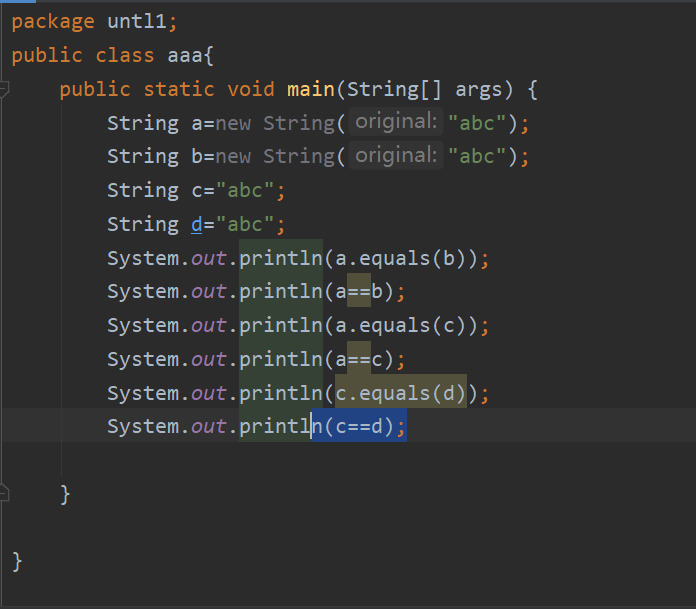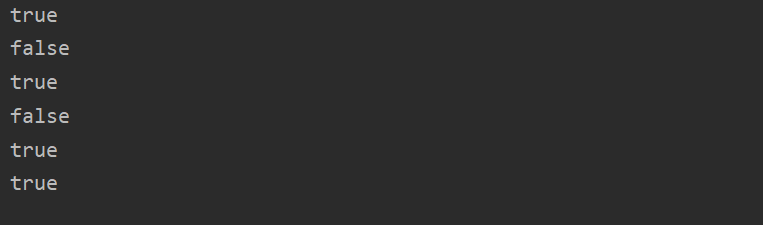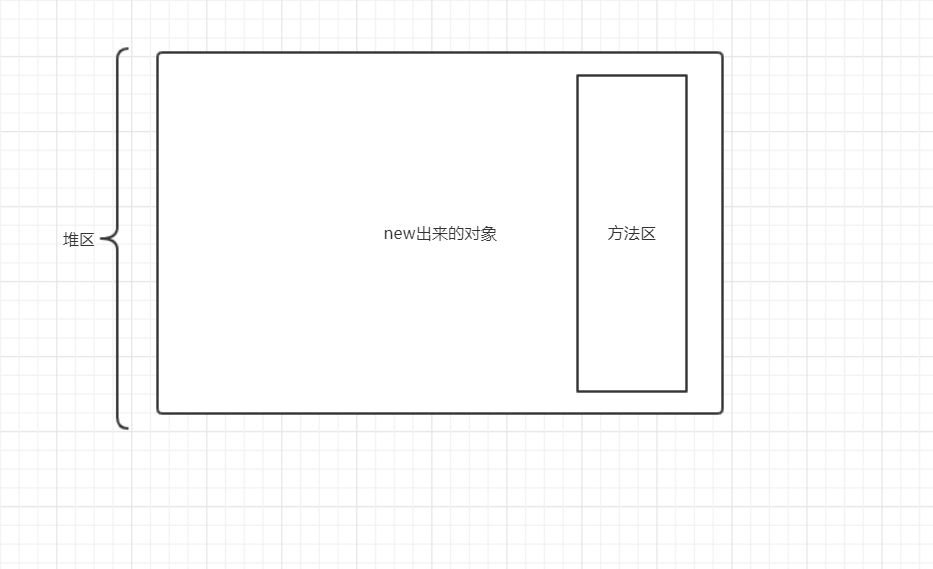1.==

2.equal

3.==和equal的区别

# 1.==

(1)我们都知道数据类型分为基本数据类型和引用数据类型，== 在比较不同的数据类型所比较的东西也不一样

# 2.equal

(1)比较基本数据类型：注意这里equal不能作为比较基本数据类型的方法
(2)比较引用数据类型：这里分为两种情况

`````` * Compares this string to the specified object.  The result is {@code
* true} if and only if the argument is not {@code null} and is a {@code
* String} object that represents the same sequence of characters as this
* object.
*
* @param  anObject
*         The object to compare this {@code String} against
*
* @return  {@code true} if the given object represents a {@code String}
*          equivalent to this string, {@code false} otherwise
*
* @see  #compareTo(String)
* @see  #equalsIgnoreCase(String)
*/
public boolean equals(Object anObject) {
if (this == anObject) {
return true;
}
if (anObject instanceof String) {
String anotherString = (String) anObject;
int n = value.length;
if (n == anotherString.value.length) {
char v1[] = value;
char v2[] = anotherString.value;
int i = 0;
while (n-- != 0) {
if (v1[i] != v2[i])
return false;
i++;
}
return true;
}
}
return false;
}
``````

(3)我们知道String在创建对象的时候可以用new也可以不用new直接赋值

``````String  a=new String("abc");
String  b="abc";
``````